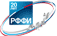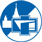Российский фонд
фундаментальных
исследованийФизический факультет
МГУ им. М.В.Ломоносова

# 08.14 Авиационная акустика

Yang Liu, Huang Jun, Yi Mingxu, Zhang Chaopu, Xiao Qian «A numerical study of the effects of design parameters on the acoustics noise of a high efficiency propeller» Акустический журнал, 63, № 6, с. pp. 699-710 (2017)

A numerical study of a high efficiency propeller in the aerodynamic noise generation is carried out. Based on RANS, three-dimensional numerical simulation is performed to obtain the aerodynamic performance of the propeller. The result of the aerodynamic analysis is given as input of the acoustic calculation. The sound is calculated using the Farassat 1A, which is derived from Ffowcs Williams–Hawkings equation, and compared with the data of wind tunnel. The propeller is modified for noise reduction by changing its geometrical parameters such as diameter, chord width and pitch angle. The trend of variation between aerodynamic analysis data and acoustic calculation result are compared and discussed for different modification tasks. Meaningful conclusions are drawn on the noise reduction of propeller.

Акустический журнал, 63, № 6, с. pp. 699-710 (2017) | Рубрика: 08.14

Li Hui «Streamline Computation Study on Rotation Aerodynamic Noise Prediction of Cross-flow Fan» Акустический журнал, 65, № 4, с. pp. 418-431 (2019)

The approach of resolving analysis the cross-flow relative motion streamline on the cross-flow straight blade is presented to predict the rotation aerodynamic noise performance of cross-flow fan by solving the dipole source term of Ffowcs Williams and Hawkings equation, while the cross-flow fan is applied in the indoor unit of split-type air-conditioner and operated in the rated condition. The calculating results of the method are respectively drawn by programming in Matlab computational language, and compared with the results of CAA numerical simulation and noise experiment. There are some differences in the distribution condition of aerodynamic force fluctuation amplitude on the blade surface, and sound pressure in the related frequency on the indoor unit casing, or on the sphere far field, between the streamline computation approach and numerical simulation. The orders of magnitudes of these calculating results solved by the analyzing streamline method are similar with that attained by the numerical simulation. The resolving analysis approach has the characteristics of decreasing the computing cost and not constructing the acoustics grid model of fan, compared with the numerical simulation. The error between the result of numerical simulation and noise test is larger than that between the result of theoretical calculation and noise test, so the approach could be used for the rotation aerodynamic noise analysis of the cross-flow fan.

Акустический журнал, 65, № 4, с. pp. 418-431 (2019) | Рубрика: 08.14